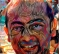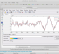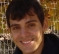(So far, 219 people got it right out of 438 for a success rate of 50%)

(Thank you to Rick Lyons, author of the top-selling book Understanding Digital Signal Processing, for submitting this Quiz Question.)

Let's say a DSP practitioner performs a forward discrete Fourier transform (DFT), zero padding, and inverse DFT processing shown in the following figure.The x(n) input is a 4-sample negative-frequency complex exponential sequence, whose frequency is 1/4th its sample rate, defined by:

x(n) = [1+j, 1-j, -1-j, -1+j].

Quiz Question: The y(n) sequence will be

Pick one:
A negative-frequency complex-valued exponential sequence.
A positive-frequency complex-valued exponential sequence.
A real-valued sinusoidal sequence.
None of the above.

[ - ]
Comment by November 10, 2019My Matlab routines:

function [Xk] = dfs(xn, N)

% Xk = DFS coeff. array over 0 <= k <= N-1

% xn = One period of periodic signal over 0 <= n <= N-1

% N = Fundamental period of xn

%

n = [0:1:N-1];

% row vector for n

k = [0:1:N-1];

% row vecor for k

WN = exp(-i*2*pi/N);

% Wn factor

nk = n'*k;

% creates a N by N matrix of nk values

WNnk = WN .^ nk;

% DFS matrix

Xk = xn * WNnk;

****************************************

function [xn] = idfs(Xk,N)

% Computes Inverse Discrete Fourier Series

% ----------------------------------------

% [xn] = idfs(Xk,N)

% xn = One period of periodic signal over 0 <= n <= N-1

% Xk = DFS coeff. array over 0 <= k <= N-1

% N = Fundamental period of Xk

%

n = [0:1:N-1];

% row vector for n

k = [0:1:N-1];

% row vecor for k

WN = exp(-i*2*pi/N);

% Wn factor

nk = n'*k;

% creates a N by N matrix of nk values

WNnk = WN .^ (-nk);

% IDFS matrix

xn = (Xk * WNnk)/N;

% row vector for IDFS values

**************************************************

xn=[Xk,zeros(1,N)]

*************************************************

function plotdfs(Xk)

%plot

N=length(Xk);

k=-N/2:N/2;

magXk = abs([Xk(N/2+1:N) Xk(1:N/2+1)]); % DFS magnitude

plot(k,magXk);

axis([-N/2,N/2,-0.5,5.5]);

xlabel("k"); ylabel("Xtilde(k)")

title("discrete Fourier transform (DFT) ")

******************************************************

my command lines:

X=[1+i, 1-i,-1-j,-1+i]

Xm=dfs(x,4)

stem(0:23,real(y))

stem(0:23,imag(y))

dfsy=dfs(y,24)

plotdfs(dfsy)

[ - ]
Comment by October 31, 2019I did it first :)

[ - ]
Comment by October 31, 2019Keep 'em coming!  Fun! Thanks, Rick Lyons!

[ - ]
Comment by November 1, 2019I got it wrong first. It was a trick question. I failed to recognize that zero padding was happening to the FOLDED spectrum thus all negative frequency components got set to zero by the zero padding. Good one. It would have been fun to also show that by prepending with zeros instead of appending would have set all positive frequencies to zero and the result would have been a negative frequency complex exponential sequence
[ - ]
Comment by November 1, 2019Yip, I fell down that rabbit hole too - I saw pad and just assumed it meant what I expected it to mean and not the 'append' that is clearly illustrated in the problem.  Next time I'll be more careful...nah, I'll just make some other silly mistake.

Score one for Mr. Lyons for the subtlety of this one.

[ - ]
Comment by November 3, 2019Hi bbhattac. "Trick question"!!  Who, me? Surely you're joking. :-)

(Actually, my Quiz Question originated from an e-mail I received recently from a young DSPer asking for help. The e-mailer was trying to perform time-domain interpolation by way of frequency-domain zero padding (more correctly called "zero stuffing").

[ - ]
Comment by November 1, 2019Great one.

If you look at the mathematical expression for the IDFT, you could make the case that based on the formula, all the terms of the DFT represent a counter-clockwise rotating phase in time (positive frequency). In fact, all the terms are equally either positive frequencies OR negative frequencies given the circular nature of the DFT.

For example, one could argue that the original sequence in frequency [0 0 0 1] can also be a positive frequency exponential. Each of the n terms of the DFT (n being 0, 1,2, ...N-1, where N is the length of the DFT) represents either a positive frequency exponential, rotating n times counter-clockwise around the unit circle OR identically, rotating N-n time clock-wise around the unit circle. The DFT sequence [0 0 0 4] represents a phasor in time with magnitude 4 and starting angle 0 rotating 3 times around the unit circle in all cases of N = 4 or greater. When N =4 as in this case, the sequence [0 0 0 4] equally represents a phasor rotating 4-3 = 1 times clockwise around the unit circle. As we append zeros, we interpolate more samples of this same underlying time domain function; 3 rotations counter-clockwise. However in all these cases of adding zeros, there is still an equivalent negative frequency: If I add 20 zeros for N = 24, then the time domain function is a phasor rotating 3 times counter-clockwise (positive frequency) OR rotating 24-3 = 21 times clockwise (negative frequency)!

(This is similar to the equivalence of rotating clockwise 270° between 2 samples, or rotating counter-clockwise 90° - the result is the same - so given it is a sampled system with a many to one result, you can't really say it is rotating one way or the other).

[ - ]
Comment by November 3, 2019Hi DanBoschen.

Although I didn't state it specifically (and it looks like I should have), the assumption with my original x(n) sequence is that it was sampled at a sample rate that satisfies the Nyquist Criterion. In which case x(n)'s complex-valued samples cannot rotate more than ±180 degrees from one sample to the next sample.

When that criterion is satisfied then there's no frequency ambiguity associated with x(n). The frequency of x(n) is negative 1/4th the sample rate.

[ - ]
Comment by November 3, 2019What is a forward FFT?

[ - ]
Comment by November 5, 2019A non inverse FFT.
[ - ]
Comment by March 21, 2020it mean fft not inverse FFT :) if tell FFT may be better

[ - ]
Comment by March 21, 2020How to plot such a plot in matlab? for this quiz maybe usefull too.

[ - ]
Comment by March 30, 2020@sh_honest: You can use plot3 function in matlab.

[ - ]
Comment by April 14, 2020thanks yes i did it.

To post reply to a comment, click on the 'reply' button attached to each comment. To post a new comment (not a reply to a comment) check out the 'Write a Comment' tab at the top of the comments.